Interactive Control Systems Tools
Help and Hints
System overview

A brief introduction to Matlab and the Interactive Control Systems Tools is presented here.

Importing data

The Interactive Control Systems Tools are created using MATLAB language and run in MATLAB environment. Because of this the data input is based on the data typing rules of the MATLAB. Since this screen is intended to show only the data typing needed for the Interactive Control Systems Tools, for detailed description about MATLAB, MATLAB environment, programming etc. please refer to the MATLAB documentation.

Typing numbers in MATLAB

For convenience entering numbers is described together with entering matrices.

Typing matrices

The following conventions explain a matrix input.

• Separate the elements of a row with blanks or commas.
• Use a semicolon, ; , to indicate the end of each row.
• Surround the entire list of elements with square brackets, [ ].

(Example 1)

If you enter A=[1 2 3;4 5 6;7 8 9] followed by Enter key, the MATLAB displays

A=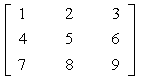(Example 2)

Input like  presents an 1x1 matrix.

(Example 3)

Input of [ ] is for an empty matrix.

Operators

The operators and precedence rules are

 + Addition - Substraction * Multiplication .* Element-by-element multiplication ./ Element-by-element division \ Element-by-element right division

Additionally, calculations as shown in the table below are possible:

 [matrix_name]^number Matrix power Example: A^2 sqrtm(number or matrix) Matrix square root Example: sqrtm(A) abs(number or matrix) Absolute value Example: abs(A) log10(number or matrix) Decimal logarithm Example: log10(A) log(number or matrix) Natural logarithm Example: log(A) exp (number or matrix) Exponential Example: exp 10 or exp A i or j Imaginary unit Example: 2+4j pi The 'pi' (3.1415926...) Example: 2*pi inf Infinite value Example: inf inv (matrix) Matrix inverse Example: inv(A) cos(number) Cosine function Example: cos (pi) sin(number) Sine function Example: sin (pi) tan(number) Tangent Example: tan (pi)

Additionally, there exist many other calculus functions, but because they are not applicable to the Interactive Control Systems Tools they are omitted here. Those interested in detailed description about MATLAB, MATLAB environment, programming etc. please refer to the MATLAB documentation.

(Example 1)

In put of 1*2*3 produces 6.

(Example 2)

It is possible to include calculus functions inside the input. For example, the input of

[-1.2 3+4;5*6 log(7)]

produces the following matrix: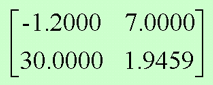Colon (:) operator

The colon operator is often used in MATLAB. It occurs in several different forms. It expresses a repeat action in the following meaning:

Definition:

 [a:b] [a a+1 a+2 ... b] generates a vector with elements between numbers 'a' and 'b', where a>b and the difference between two neighboring elements is 1. (If a

(Example 1)

Typing

[ 1 : 3 ; 2 : 4 ; 3 : 5 ]

produces a matrix like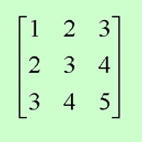(Example 2)

Input as

[ 1:0.5:3 ; 2.6:0.1:3 ]

produces the following matrix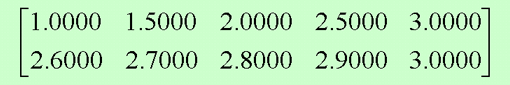A brief introduction of the Interactive Control Systems Tools

Running the tools

When the tools are started the following main menu appears inside the browser window: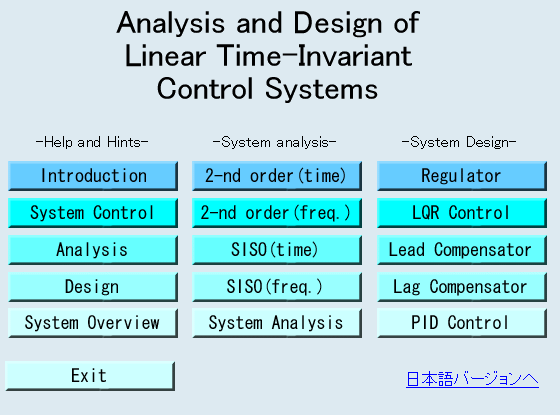• Depending on MATLAB's "Language" property the display language is decided automatically. For instance, if he "language" property is Japanese then an opening screen written in Japanese is displayed. Otherwise, English window is used.

At present, the main window has three columns of buttons: 1)Help and Hints, 2) System Analysis, and 3) System Design. Clicking a button produces a tool-window for learning a topic in basic control theory.

Learning environment

All tool-windows have similar construction and can be used easily and intuitively. The tool-window "pattern" looks like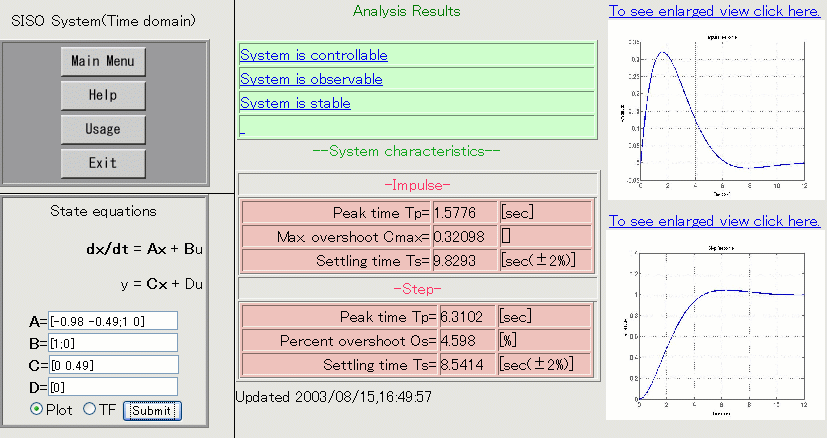Every window has four areas. Area (1) is a set of four buttons called System buttons, as explained below. Area (2) is the data input area, in area (3) graphical results are shown, and area (4) is for displaying results from the system analysis. These areas are briefly described below.

(1) System buttons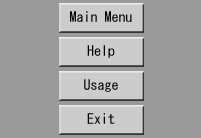"Main Menu" button closes the current tool and invokes back the main menu. As a result of clicking the "Help" button a help window about the basic theory in the current tool. The "Usage" button is for explanation about the manipulations inside the current tool window. Usually, there is no need to read this help, but it is supplied for completeness. "Exit" is for quitting from the Interactive Control Systems Tools.

(2) Data input area

In the data input area the necessary parameters for every tool are typed. (In the picture below the Data input area for one of the tools is shown.)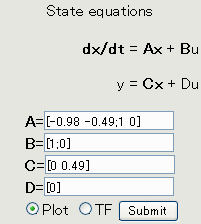In every tool, inside this area there is a "Submit" button for invoking the visual results or sending over the internet the description of the system to be analyzed or designed. Additionaly in some tools there are radiobuttons for selecting what kind of job will be performed after clicking the "Submit" button. For example, in the above picture there are two radio buttons: the first one ("Plot" radio button) is for plotting the results of simulation, and when the second one ("TF" button) is selected a frame for inputting the transfer function of the system will appear.

(3) Graphical Results

The contents of this area is generated (regenerated) when "Submit" is clicked.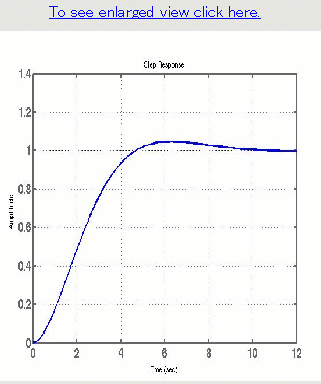Clicking the picture an emlarged and more detailed graph is displsyed in separate browser window.

(4) System analysis Results

Here, error messages (in a case of wrong input data) or results from the analysis of the system are shown as brief messages inside one or several links.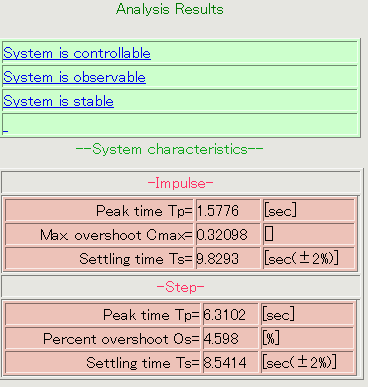By clicking these links a help screen with necessary explanations is displayed.